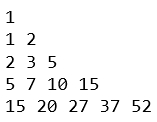### Program to generate Pattern/Pyramid in java - Pyramid 14

Write a program to generate this Pattern.We will be using jagged array to generate such pyramid.

Program to generate above pattern >
 /** JavaMadeSoEasy.com */ public class Pyramid14 {    public static void main(String... args) {           int ar[][] = new int[]; //jagged array           int start = 0;           int prev = 0;           int sum = 1; // keep initial sum as 0           for (int i = 0; i < ar.length; i++) {                  ar[i] = new int[i + 1];                  prev = 0;                  for (int j = 0; j < ar[i].length; j++) {                        if (i > 0 && j > 0) {                               prev = ar[i][j - 1];                               start = ar[i - 1][j - 1];                               sum = start + prev;                               ar[i][j] = sum;                        } else {                               ar[i][j] = sum;                        }                        System.out.print(sum + " ");                  }                  start = sum; // assign sum to start                  System.out.println(); // for new line           } // end for loop    } } /* 1 1 2 2 3 5 5 7 10 15 15 20 27 37 52 */

Having any doubt? or you you liked the tutorial! Please comment in below section.